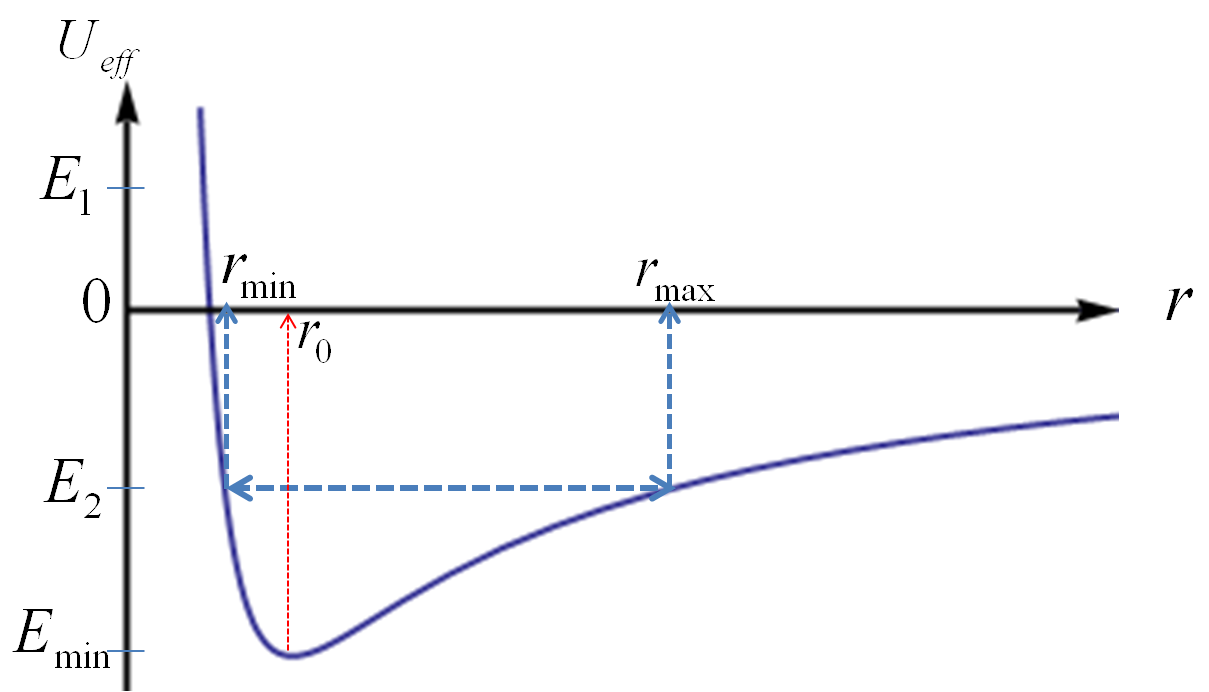## Section12.6Energy Conservation

###### Two Bodies Interacting with Gravitation.

Since gravitational force is a conservative force, the total energy of the two-body interacting via the gravitational force is conserved. The total energy $E$ of the two-body system is given by the following.

\begin{equation} E = K + U = \left(\dfrac{1}{2}m_1v_1^2 + \dfrac{1}{2}m_2v_2^2 \right) - G_N\dfrac{m_1m_2}{r}.\tag{12.6.1} \end{equation}

The kinetic energy part can also be written as sum of two parts, the kinetic energy of the center of mass and the kinetic energy of the motion with respect to the center of mass.

\begin{equation} E = \left(\dfrac{1}{2}M V_\text{cm}^2 + \dfrac{1}{2}\mu v_\text{rel}^2 \right) - G_N\dfrac{m_1m_2}{r},\label{eq-gravity-energy-cons-cm-and-rel-regular}\tag{12.6.2} \end{equation}

where

\begin{align*} \amp V_\text{cm} = \text{ Speed of CM},\\ \amp v_\text{rel} = \text{ Speed of }m_1\text{ relative to }m_2, \\ \amp M = m_1 + m_2,\\ \amp \mu = \dfrac{m_1 m_2}{m_1 + m_2}. \end{align*}

Often we work in a coordinate system whose origin is fixed at the CM. In this system, $V_\text{cm}=0\text{.}$ Then, we have only the relative position between the two particles to study.

\begin{equation} E = \dfrac{1}{2}\mu v_\text{rel}^2 - G_N\dfrac{m_1m_2}{r}\ \ (\text{CM Frame})\label{eq-gravity-energy-cons-cm-and-rel-cmframe}\tag{12.6.3} \end{equation}

### Subsection12.6.1(Calculus) Effective Potential Energy

Since gravitational force is spherically symmetric, it makes sense to use sherical coordinates. For planar motion of planets around the Sun, we need only polar coordinates. In polar coordinates, the energy in the CM frame, which will have $V_\text{cm}=0\text{,}$ is

\begin{equation} E = \dfrac{1}{2}\mu v_r^2 + \dfrac{1}{2}\mu r^2 \omega^2 - G_N\dfrac{m_1m_2}{r},\tag{12.6.4} \end{equation}

where $r$ is the relative distance between the particles, $v_r$ radial speed, and $\omega$ speed for the angular variable $\theta$ of the polar coordinate in the plane of motion,

\begin{equation*} v_r = \dfrac{dr}{dt},\ \ \text{ and }\ \ \omega = \dfrac{d\theta}{dt}. \end{equation*}

The angular part can be expressed in terms of conserved angular momentum,

\begin{equation} l = \mu \omega r^2 \ \ \longrightarrow\ \ \omega = \dfrac{l}{\mu r^2}.\tag{12.6.5} \end{equation}

After expressing $\omega$ in terms of $l\text{,}$ we can group together non-radial-velocity terms.

\begin{equation} E = \dfrac{1}{2}\mu v_r^2 + \left( \dfrac{1}{2} \dfrac{l^2}{\mu r^2} - G_N\dfrac{m_1m_2}{r} \right).\tag{12.6.6} \end{equation}

The quantity within parenthesis, $( \cdots )$ is called effective potential energy, and denoted by $U_\text{eff}\text{.}$ With

\begin{equation} U_\text{eff} = \dfrac{1}{2} \dfrac{l^2}{\mu r^2} - G_N\dfrac{m_1m_2}{r},\label{eq-grav-ener-cons-ueff}\tag{12.6.7} \end{equation}

we write energy more simply as

\begin{equation} E = \dfrac{1}{2}\mu v_r^2 + U_\text{eff} \equiv K_r + U_\text{eff},\label{eq-grav-ener-cons-kr-ueff}\tag{12.6.8} \end{equation}

where $K_r$ is kinetic energy in the radial motion only. When we write energy this way, the problem looks like the problem of one variable $r\text{,}$ which can vary in range $[0,\infty)\text{.}$ Of course, in the real world, the planet is moving in a plane with both the radial coordinate and the angular coordinate. Equation (12.6.8) helps us focus on the radial motion of reduced particle $\mu$ alone.

### Subsection12.6.2Interpreting Effective Potential Energy

How to use effective potential energy in Eq. (12.6.7) and (12.6.8)? First of all, we note the $K_r \ge 0$ since $\mu$ and square of speed are positive real numbers. Therefore

\begin{equation*} E - U_\text{eff} \ge 0, \text{ for all }r. \end{equation*}

To explore $U_\text{eff}$ further, let us use a simpler notation.

\begin{equation} U_\text{eff} = \dfrac{1}{2} \dfrac{l^2}{\mu r^2} - G_N\dfrac{m_1m_2}{r} \Leftrightarrow \dfrac{\alpha}{r^2} - \dfrac{\beta}{r},\tag{12.6.9} \end{equation}

with

\begin{equation*} \alpha = \dfrac{l^2}{2 \mu},\ \ \beta = G_Nm_1m_2. \end{equation*}

Figure 12.6.1 shows $U_\text{eff}\text{ vs }r\text{.}$ The figure shows four distinct energy values of interest, $E=0,\, E_1,\, E_2,$ and $E_\text{min}=U_0\text{.}$ We can see the turning points represented by these energy values by solving $E = U_\text{eff}\text{,}$ we will have the values of $r$ for turning points in the motion in relative position, since at those values we will have $K_r=0\text{,}$ i.e., $v_\text{r}=0\text{.}$

\begin{equation} E r^2 + \beta r - \alpha = 0.\tag{12.6.10} \end{equation}

This equation has following solutions.

\begin{align} \amp r = \dfrac{\alpha}{\beta} \ \ (E=0), \tag{12.6.11}\\ \amp r = \dfrac{1}{E} \left(-\beta + \sqrt{ \beta^2 + 4\alpha E } \right)\ \ (E \gt 0), \tag{12.6.12}\\ \amp r = \dfrac{1}{|E|} \left(\beta \pm \sqrt{ \beta^2 - 4\alpha |E| } \right)\ \ (E \lt 0), \tag{12.6.13} \end{align}

where we need to make sure that

\begin{equation*} \beta^2 \ge 4 \alpha |E|. \end{equation*}

The solution for $E=0$ has one turning point with orbit having the shape of a parabola. The solution for $E \gt 0$ has also only one turning point with the orbit a hyperbola.

The two solutions in the case of $E \lt 0$ refers to the perihelion and aphelion of a planet in the planet/Sun system.

\begin{align} r_\text{min} \amp = \dfrac{1}{|E|} \left(\beta - \sqrt{ \beta^2 - 4\alpha|E| } \right)\ \ (E\lt 0,\ \ |E| \lt \beta^2/4\alpha) \tag{12.6.14}\\ r_\text{max} \amp = \dfrac{1}{|E|} \left(\beta + \sqrt{ \beta^2 - 4\alpha|E| } \right)\ \ (E\lt 0,\ \ |E| \lt \beta^2/4\alpha) \tag{12.6.15} \end{align}

The solutions of orbit equation for these conditions show that the orbit is ellipse with the smallest distance between the masses given by $r_\text{min}$ and the largest distance $r_\text{min} \text{.}$ When $(E\lt 0,\ \ |E| = \beta/4\alpha)\text{,}$ there is only one solution with

\begin{equation*} r_0 = \dfrac{2a}{\beta}\ \ (E\lt 0,\ \ |E| = \beta^2/4\alpha), \end{equation*}

which is the radius of the circle of motion of the relative position.Figure 12.6.1. Plot of effective potential energy versus radial distance $r\text{.}$ Four energy levels of interest are indicated on the axis. They are $E = 0,\ E_1,\ E_2, E_\text{min}\text{.}$ The system cannot have energy less than the minimum of $U_\text{eff}$ since that would mean $K_r\lt 0\text{,}$ which is impossible.